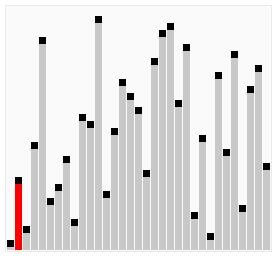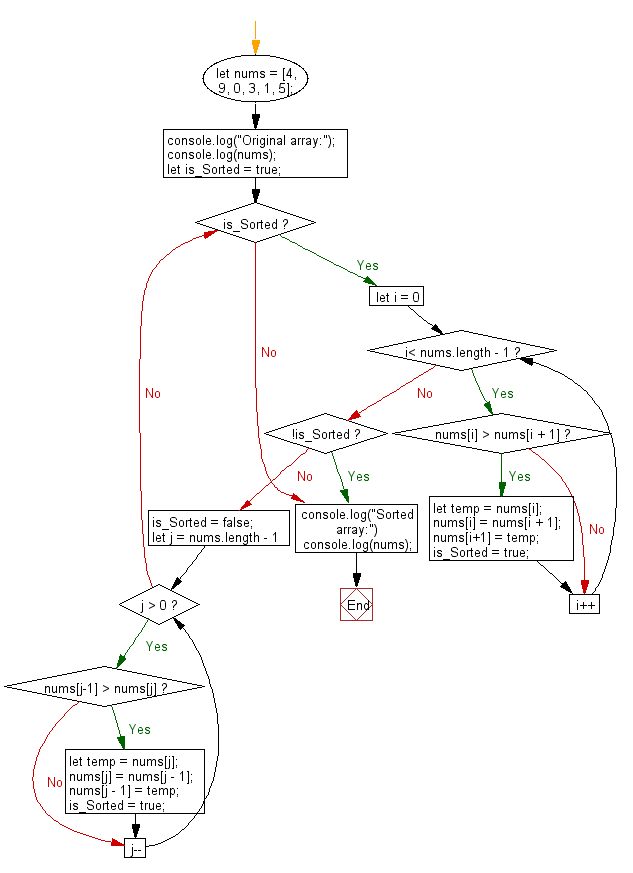# JavaScript Sorting Algorithm: Cocktail shaker sort

## JavaScript Sorting Algorithm: Exercise-8 with Solution

Write a JavaScript program to sort a list of elements using Cocktail shaker sort.

Cocktail shaker sort (also known as bidirectional bubble sort, cocktail sort, shaker sort, ripple sort, shuffle sort, or shuttle sort ) is a variation of bubble sort that is both a stable sorting algorithm and a comparison sort. The algorithm differs from a bubble sort in that it sorts in both directions on each pass through the list. This sorting algorithm is only marginally more difficult to implement than a bubble sort, and solves the problem of turtles in bubble sorts. It provides only marginal performance improvements, and does not improve asymptotic performance; like the bubble sort, it is not of practical interest,, though it finds some use in education.

Visualization of shaker sort :Sample Solution: -

HTML Code:

``````<!DOCTYPE html>
<html>
<meta charset="utf-8">
<title>JavaScript program of Cocktail sort</title>
<body></body>
</html>
```
```

JavaScript Code:

``````let nums = [4, 9, 0, 3, 1, 5];
console.log("Original array:");
console.log(nums);
let is_Sorted = true;
while (is_Sorted){
for (let i = 0; i< nums.length - 1; i++){
if (nums[i] > nums[i + 1])
{
let temp = nums[i];
nums[i] = nums[i + 1];
nums[i+1] = temp;
is_Sorted = true;
}
}

if (!is_Sorted)
break;

is_Sorted = false;

for (let j = nums.length - 1; j > 0; j--){
if (nums[j-1] > nums[j])
{
let temp = nums[j];
nums[j] = nums[j - 1];
nums[j - 1] = temp;
is_Sorted = true;
}
}
}
console.log("Sorted array:")
console.log(nums);
```
```

Sample Output:

```Original array:
[4,9,0,3,1,5]
Sorted array:
[0,1,3,4,5,9]
```

Flowchart:Live Demo:

See the Pen searching-and-sorting-algorithm-exercise-8 by w3resource (@w3resource) on CodePen.